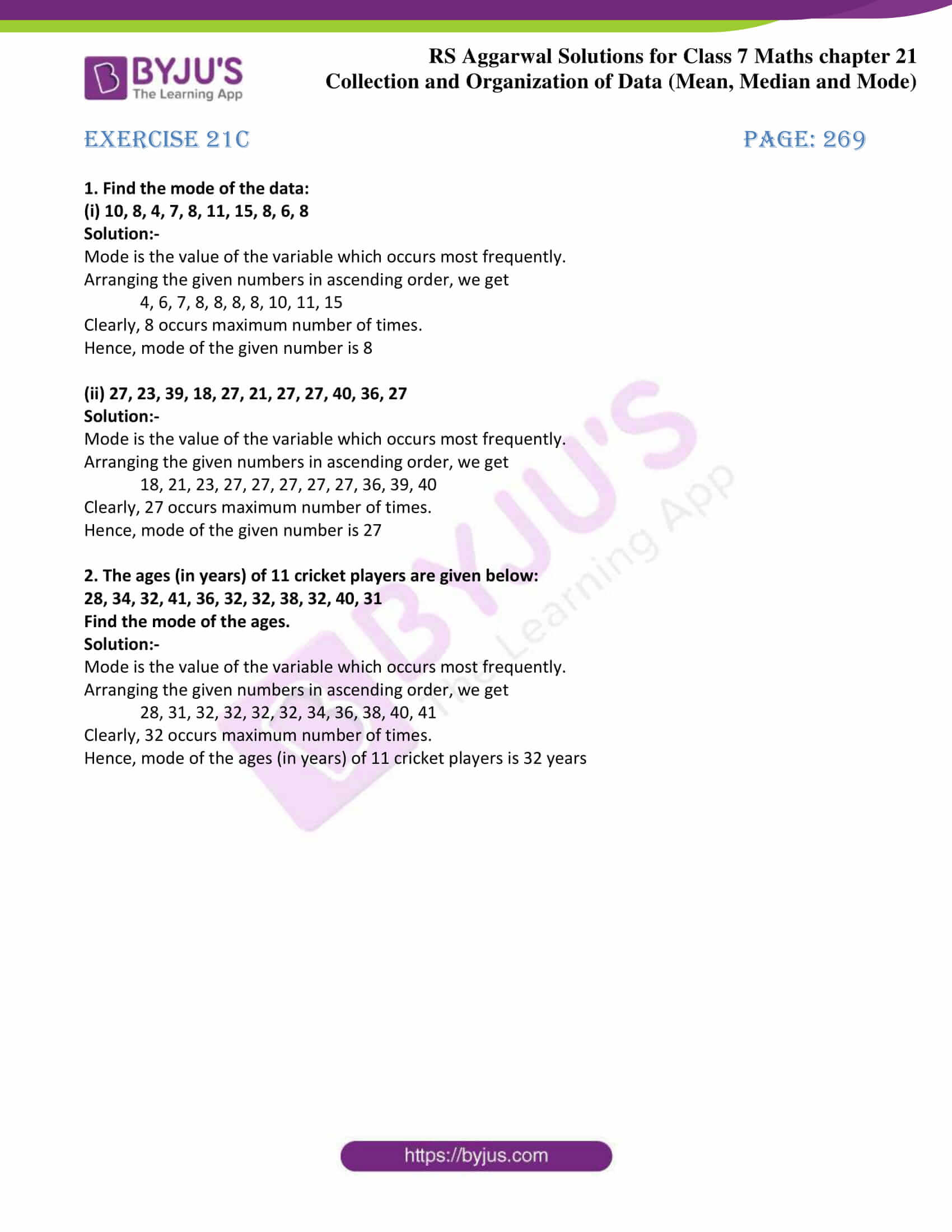# RS Aggarwal Solutions for Class 7 Maths Exercise 21C Chapter 21 Collection and Organisation of Data (Mean, Median and Mode)

RS Aggarwal Solutions for Class 7 Maths Exercise 21C Chapter 21 Collection and Organisation of Data (Mean, Median and Mode) in PDF are given here. Students will go through this RS Aggarwal Solutions for Class 7 Maths Exercise 21C Chapter 21 thoroughly with more practice. Mode of ungrouped data is the only topic covered in this exercise. A mode is defined as the value of the variable which occurs most frequently. Students can score good marks in Maths by practising RS Aggarwal Class 7 Solutions.

## Download the PDF of RS Aggarwal Solutions For Class 7 Maths Chapter 21 Collection and Organisation of Data (Mean, Median and Mode) – Exercise 21C### Access answers to Maths RS Aggarwal Solutions for Class 7 Chapter 21 – Collection and Organisation of Data (Mean, Median and Mode) Exercise 21C

1. Find the mode of the data:

(i) 10, 8, 4, 7, 8, 11, 15, 8, 6, 8

Solution:-

Mode is the value of the variable which occurs most frequently.

Arranging the given numbers in ascending order, we get

4, 6, 7, 8, 8, 8, 8, 10, 11, 15

Clearly, 8 occurs maximum number of times.

Hence, mode of the given number is 8

(ii) 27, 23, 39, 18, 27, 21, 27, 27, 40, 36, 27

Solution:-

Mode is the value of the variable which occurs most frequently.

Arranging the given numbers in ascending order, we get

18, 21, 23, 27, 27, 27, 27, 27, 36, 39, 40

Clearly, 27 occurs maximum number of times.

Hence, mode of the given number is 27

2. The ages (in years) of 11 cricket players are given below:

28, 34, 32, 41, 36, 32, 32, 38, 32, 40, 31

Find the mode of the ages.

Solution:-

Mode is the value of the variable which occurs most frequently.

Arranging the given numbers in ascending order, we get

28, 31, 32, 32, 32, 32, 34, 36, 38, 40, 41

Clearly, 32 occurs maximum number of times.

Hence, mode of the ages (in years) of 11 cricket players is 32 years

### Access other exercises of RS Aggarwal Solutions For Class 7 Chapter 21 – Collection and Organisation of Data (Mean, Median and Mode)

Exercise 21A Solutions

Exercise 21B Solutions